# Seemingly Unrelated Regression Model using Gibbs Sampler

### Home | Academic Articles

Purpose

The purpose of the seemingly unrelated regression (SUR) model using Gibbs sampler is to create a model that has a better fit than the corresponding model under the Frequentist school.

Example

Let’s suppose we have 3 sets of data to create 3 models.

Here is the first data set:

 y 2.1 4.5 6.8 9.4 10.8 X1 1 2 3 4 5

And the second:

 y 4.8 9.6 13.7 19.6 21.4 X2 1 2 3 4 5 X3 0.25 0.42 0.84 1.32 1.64

And the third:

 y 0.5 1.1 1.4 2.3 3.4 X4 10.2 20.5 30.4 41.6 50.2

Using least squares, the summary statistics for each of the coefficients are:

Model #1

 Coefficients Standard Error t Stat P-value Lower 95% Upper 95% Intercept 0.03 0.3728 0.0805 0.9409 -1.1564 1.2164 X1 2.23 0.1124 19.8402 0.0003 1.8723 2.5877

Model #2

 Coefficients Standard Error t Stat P-value Lower 95% Upper 95% Intercept 1.1418 2.1708 0.5260 0.6514 -8.1986 10.4822 X2 3.8261 2.9894 1.2799 0.3290 -9.0363 16.6886 X3 1.3420 8.0463 0.1668 0.8829 -33.2786 35.9626

Model #3

 Coefficients Standard Error t Stat P-value Lower 95% Upper 95% Intercept -0.3674 0.3177 -1.1562 0.3313 -1.3786 0.6438 X4 0.0689 0.0094 7.3223 0.0053 0.0390 0.0989

If all the variables are combined into one model, this is the result:

 Coefficients Standard Error t Stat P-value Lower 95% Upper 95% Intercept 0.0492 0.4615 0.1066 0.9172 -0.9791 1.0775 x1 2.2248 0.1557 14.2931 0.0000 1.8779 2.5716 x2 5.1036 0.9861 5.1753 0.0004 2.9064 7.3009 x3 -1.8103 2.9568 -0.6122 0.5540 -8.3984 4.7778 x4 0.0577 0.0153 3.7725 0.0036 0.0236 0.0918

The goal is to create models that solve for the coefficients simultaneously. Arnold Zellner introduced a concept called the seemingly unrelated regression (SUR) model.

As explained in the technical details, the Gibbs sampler was run for 50,000 iterations.

If one has two models, the one that has the lower sum of squared errors is the model with the better fit.

In regard to the sum of the squared errors, these are the results:

 SUR Model #1 Model #2 Model #3 Frequentist 4.0339 0.379 3.3770 0.2719 4.6119

The sum of squared errors is less for each of the individual models than for the SUR model which, in turn, is less than that of the Frequentist model. It should be noted that when the Gibbs sampler is run for 100,000 iterations, the sum of squared errors goes down to 4.0331 which would seem to suggest that running the extra 50,000 iterations is not worth the while.

Technical details

In the SUR model, this is how the data would be set up:

 y1 x1 x2 x3 x4 2.1 1 0 0 0 4.5 2 0 0 0 6.8 3 0 0 0 9.4 4 0 0 0 10.8 5 0 0 0 4.8 0 1 0.25 0 9.6 0 2 0.42 0 13.7 0 3 0.84 0 19.6 0 4 1.32 0 21.4 0 5 1.64 0 0.5 0 0 0 10.2 1.1 0 0 0 20.5 1.4 0 0 0 30.4 2.3 0 0 0 41.6 3.4 0 0 0 50.2

Of course, for each model, you would still need a vector of 1s for the intercept, which leads to this matrix which I call the X new matrix:

 y1 x10 x11 x20 x21 x22 x30 x31 2.1 1 1 0 0 0 0 0 4.5 1 2 0 0 0 0 0 6.8 1 3 0 0 0 0 0 9.4 1 4 0 0 0 0 0 10.8 1 5 0 0 0 0 0 4.8 0 0 1 1 0.25 0 0 9.6 0 0 1 2 0.42 0 0 13.7 0 0 1 3 0.84 0 0 19.6 0 0 1 4 1.32 0 0 21.4 0 0 1 5 1.64 0 0 0.5 0 0 0 0 0 1 10.2 1.1 0 0 0 0 0 1 20.5 1.4 0 0 0 0 0 1 30.4 2.3 0 0 0 0 0 1 41.6 3.4 0 0 0 0 0 1 50.2

In the frequentist school, the beta vector β and the covariance matrix of the error terms ∑ are fixed quantities. However, in the Bayesian school, β follows a normal distribution and ∑ follows an inverse Wishart distribution.

This is the basic way the Gibbs sampler works for the SUR model: Start off with least squares as in the frequentist school. From that, you create the E matrix of the error terms. Since there are 3 models and each model has 5 rows, the E matrix is a 5x3 matrix. For the above models, this would be the E matrix:

 -0.16 -0.504 0.1645 0.01 0.2414 0.0546 0.08 -0.0492 -0.3276 0.45 1.3795 -0.1994 -0.38 -1.0767 0.3079

From that, you create the S matrix which is E’E. For the above models, this would be the S matrix:

 0.379 1.109 -0.2587 1.109 3.3771 -0.6602 -0.2587 -0.6602 0.2719

It should be noted that the diagonal elements are equivalent to the sum of squared errors (residuals) from the regression ANOVA table for each model.

To get the sigma matrix, we divide the elements by the number of rows in each model. Since there are 5 rows, this is the sigma matrix for the above data:

 0.0758 0.2218 -0.0517 0.2218 0.6754 -0.132 -0.0517 -0.132 0.0544

As you notice from the above matrix, the error terms are correlated. However, we

would like uncorrelated errors. The way we do that is by first computing the lower Cholesky root of the sigma matrix which we call the L matrix. This would be the result:

 0.275318 0 0 0.805645 0.162314 0 -0.18795 0.119367 0.069388

We then take the inverse of the L matrix:

 3.6322 0 0 -18.0282 6.1609 0 40.8517 -10.5985 14.4117

The dimensions of the X new matrix are 15x7. However, the dimensions of L inverse matrix are 3x3. To create a 15x15 matrix, we compute the Kronecker product of the L inverse matrix and the identity matrix of dimensions 5x5, since each model has 5 rows. This is what the result looks like for the first 5 rows and columns:

 3.6322 0 0 0 0 0 3.6322 0 0 0 0 0 3.6322 0 0 0 0 0 3.6322 0 0 0 0 0 3.6322

We then create the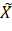matrix which is the product of the above Kronecker

product and the X new matrix.

 14.4117 14.4117 0 0 0 0 0 3.6322 7.2644 0 0 0 0 0 3.6322 10.8966 0 0 0 0 0 3.6322 14.5288 0 0 0 0 0 3.6322 18.161 0 0 0 0 0 -18.0282 -18.0282 6.1609 6.1609 1.54022 0 0 -18.0282 -36.0564 6.1609 12.3218 2.58758 0 0 -18.0282 -54.0846 6.1609 18.4827 5.17516 0 0 -18.0282 -72.1128 6.1609 24.6436 8.13239 0 0 -18.0282 -90.141 6.1609 30.8045 10.1039 0 0 40.8517 40.8517 -10.5985 -10.5985 -2.64963 14.4117 146.999 40.8517 81.7034 -10.5985 -21.197 -4.45137 14.4117 295.44 40.8517 122.555 -10.5985 -31.7955 -8.90274 14.4117 438.116 40.8517 163.407 -10.5985 -42.394 -13.99 14.4117 599.527 40.8517 204.258 -10.5985 -52.9925 -17.3815 14.4117 723.467

We can also create the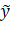vector which is the product of the Kronecker product and the y vector. This is what it looks like for this data:

 30.2646 16.3449 24.699 34.1427 39.2278 -8.2869 -21.9823 -38.1874 -48.7114 -62.8613 42.1216 97.9399 152.768 209.422 263.39

This is the point at which the Bayesian approach departs from that of the frequentist school.

We start with the prior distributions of β and ∑. To do that, we introduce some new terms.

The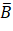vector is the average of previous beta vectors. If there are no previous beta vectors, it is a vector of 0s.

The A matrix is known as the precision matrix. To say there is a bit of literature on this matrix is a tad of an understatement. However, in a PDF file put together by Peter E. Rossi (one of the authors of Bayesian Statistics and Marketing), I found a formulation of it by multiplying the identity matrix by a scalar of 0.05. As a twist on this, I tried a scalar of the product of the number of coefficients and 0.01. Since there are 4 X variables and 3 intercepts for a total of 7 coefficients, the scalar for the A matrix would be 0.07.

The prior distribution of β follows a normal distribution with the mean equal toand the covariance matrix equal to A-1.

The V matrix is constructed by taking the sigma matrix constructed previously and multiplying it by the degrees of freedom v = n – k in which k represents the number of coefficients. Since n = 15 and k = 7, we have v = 8 degrees of freedom. For this data set, this is the V matrix:

 0.5306 1.5526 -0.3619 1.5526 4.7278 -0.924 -0.3619 -0.924 0.3808

The prior distribution of ∑ follows an inverse Wishart distribution with parameters V and v degrees of freedom.

We now combine the prior distributions and the data to create the posterior distributions.

In the frequentist school, B = (X’X)-1(X’Y). However, in the Bayesian school,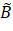= (X’X + A)-1(X’Y + A).

Onceis constructed, the posterior distribution of β follows a normal distribution with the mean ofand covariance matrix of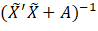.

If we assume that thevector is a vector of 0s, then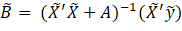.

For this data set, this is:

 -0.1569 2.27455 0.383083 4.32832 0.331196 -0.24892 0.066227

In order to generate β, we need a vector of Z values which follows a normal distribution with mean of 0 and standard deviation of 1. Since there are 7 coefficients, we need a vector of 7 Z values. Suppose, the computer generates this

vector:

 0.7839 2.5079 1.8482 -1.264 -0.2313 -0.8057 0.234

We also need the lower Cholesky root ofFor this data, the current value of this matrix is:

 0.01062 -0.00452 0.031115 -0.01332 0.00025 -0.00732 0.000305 -0.00452 0.003009 -0.01327 0.008877 -0.00019 0.003126 -0.0002 0.031115 -0.01327 0.130568 -0.06583 0.052223 -0.00036 0.000331 -0.01332 0.008877 -0.06583 0.062417 -0.09222 0.003747 -0.00042 0.00025 -0.00019 0.052223 -0.09222 0.249939 -0.00112 4.36e-05 -0.00732 0.003126 -0.00036 0.003747 -0.00112 0.026158 -0.00078 0.000305 -0.0002 0.000331 -0.00042 4.36e-05 -0.00078 3.22e-05

The lower root is:

 0.103054 0 0 0 0 0 0 -0.04386 0.032934 0 0 0 0 0 0.301925 -0.00082 0.198514 0 0 0 0 -0.12922 0.097434 -0.13465 0.134514 0 0 0 0.002426 -0.00264 0.259367 -0.42169 0.069514 0 0 -0.07106 0.000278 0.10628 0.065777 -0.01119 0.073216 0 0.002959 -0.00221 -0.00284 -0.00151 0.00186 -0.00196 0.000919

Since this is a multivariate normal distribution, we multiply the lower matrix with the Z vector and add. This is the result:

 0.7839 2.5079 1.8482 -1.264 -0.2313 -0.8057 0.234

When we multiply X and β and calculate the error terms, we can create the E matrix:

 -1.1918 4.27363 -1.0811 -1.2997 10.377 -2.8913 -1.5076 15.8381 -4.9079 -1.4155 23.1131 -6.6287 -2.5234 26.2511 -7.5411

After that, we once again create the S matrix which is E’E:

 13.7537 -141.416 40.8576 -141.416 1600.13 -463.527 40.8576 -463.527 134.424

The posterior distribution of ∑ follows an inverse Wishart distribution with parameters S + V and degrees of freedom v + n in which n is the number of rows in each model. For this data set, this is the sum of S and V:

 14.2843 -139.863 40.4957 -139.863 1604.86 -464.451 40.4957 -464.451 134.804

The degrees of freedom are 8 + 5 = 13.

To generate ∑, we need the T matrix. Since there are 3 models, the dimensions of the T matrix are 3 x 3. For this data set, this is the basic setup of the T matrix: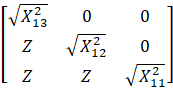For example,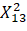is a chi-square variable with 13 degrees of freedom.

Suppose, the following T matrix is generated:

 7.20196 0 0 -0.1135 15.2643 0 0.567206 0.759346 8.22673

We also need U which is the upper Cholesky root of the S + V matrix:

 3.77945 -37.0062 10.7147 0 15.3427 -4.42824 0 0 0.624956

We then generate C = T’U:

 51.8683 -2.54992 8.66506 0 232.998 17.8378 0 0 67.679

Finally, ∑ = (C-1)(C-1)’ :

 0.000378119 3.76071e-06 -3.72939e-05 3.76071e-06 1.96999e-05 -1.6714e-05 -3.72939e-05 -1.6714e-05 0.000218319

We then use this to generate the next β and so on. After running 50,000 iterations of the Gibbs sampler, the averages of the generated β and ∑ are calculated. This is due to the random Z and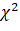values that are generated in each iteration. This is a result for β:

 Coefficient Beta (Gibbs) SE (Gibbs) Beta (LS) SE (LS) X10 0.1073 2.4016 0.03 0.3728 X11 2.2077 0.2182 2.23 0.1124 X20 1.1355 0.2255 1.1418 2.1708 X21 3.8157 0.0751 3.8261 2.9894 X22 1.3808 0.4142 1.3420 8.0463 X30 -0.3544 0.2041 -0.3674 0.3177 X31 0.0686 0.0002 0.0689 0.0094

As a comparison, the coefficients and standard errors from the individual least square models are included. It should be noted that the standard errors for models #2 and #3 under the Gibbs sampler are quite less than under least squares.

After 50,000 iterations, this is a result for ∑:

 2.18718 -0.62216 0.501253 -0.62216 0.188484 -0.13562 0.501253 -0.13562 0.183619

The aforementioned standard errors are calculated by computing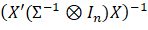In this equation, n represents the number of rows in each model, which, in this case, is 5.

Reference:

Rossi, P.E., Allenby, G.M., and McCullogh, R. Bayesian Statistics and Marketing. Chichester: John Wiley & Sons, 2005.

Zellner, A. An Introduction to Bayesian Inference in Econometrics. New York: John Wiley & Sons, 1970.